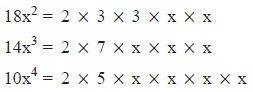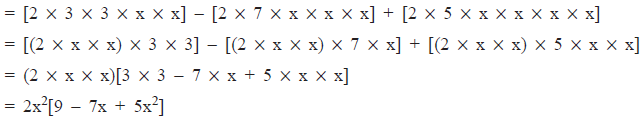Courses

# Points to Remember- Factorisation Class 8 Notes | EduRev

## Class 8 Mathematics by Full Circle

Created by: Full Circle

## Class 8 : Points to Remember- Factorisation Class 8 Notes | EduRev

The document Points to Remember- Factorisation Class 8 Notes | EduRev is a part of the Class 8 Course Class 8 Mathematics by Full Circle.
All you need of Class 8 at this link: Class 8

Points to Remember
• Factorisation means write an expression as a product of its factors.
• Like prime factors, an irreducible factor, a factor which cannot be expressed further as a product of factors.
• Some expression can easily be factorised using these identities:
I. a2 + 2ab + b2 = (a + b)2
II. a2 – 2ab + b2 = (a – b)2
III. a2 – b2 = (a – b)(a + b)
IV. x2 + (a + b)x + ab = (x + a)( x+ b)
• The number 1 is a factor of every algebraic term also, but it is shown only when needed.
• When factorisation of x2 + (a + b)x + ab is done by splitting the middle term, the two numbers which give the product ab and (a + b) as the coefficient of x have to be chosen very carefully with correct sign.

We Know That
(i) (a + b)2 = a2 + b2 + 2ab
(ii) (a – b)2 = a2 + b2 – 2ab
(iii) a2 – b2 = (a + b)(a – b)
(iv) 1 is a factor of every term of an algebraic expression. Unless it is specially required, we do not show 1 as a separate factor of any term.
(v) Factorisation means writing an expression as product of factors.

Note:
In case of factorisation of a term of an expression, the word ‘irreducible’ is used in place of ‘prime’. For example, 6pq = 2 * 3 * pq is not the irreducible form because pq can further be factorised as p q, i.e. the irreducible form of 6pq = 2 * 3 * p * q.

Example 1: Write 10y as irreducible factor form.
Solution: We have
10 = 2 * 5
xy = x *y
∴  10xy = 2 * 5* x * y

FACTORISATION USING COMMON FACTORS
Example 1: Factorise 18x2 – 14x3 + 10x4
Solution: We haveObviously, the common factors of these terms are 2, and .
∴ 18x2 – 14x3 + 10x4Thus, 18x2 – 14x3 + 10x4 = 2x2[9 – 7x + 5x2]

FACTORISATION BY REGROUPING TERMS
In certain cases the given expression cannot be factorised easily but by rearranging its terms, we can form groups leading to factorization.
Eample 1: Factorise 9x + 18y + 6xy + 27
Solution: Here, we have a common factor 3 in all the terms.
∴ 9x + 18y + 6xy + 27 = 3[3x + 6y + 2xy + 9]
We find that 3x + 6y = 3(x + 2y) and 2xy + 9 = 1(2xy + 9)
i.e. a common factor in both the groups does not eist,
Thus, 3x + 6y + 2xy + 9 cannot be factorised.
On regrouping the terms, we have
3x + 6y + 2xy + 9 = 3x + 9 + 2xy + 6y
= 3(x + 3) + 2y(x + 3)
= (x + 3)(3 + 2y)
Now, 3[3x + 6y + 2xy + 9] = 3[(x + 3)(3 + 2y)]
Thus, 9x + 18y + 6xy + 27 = 3(x + 3)(2y + 3)

Solved Examples:
Q1: Let f(x)=2x3+16x2+44x+42 be a polynomial having one of the factors as (x2+5x+7), then the other factor of f(x) would be a multiple of:
A) 1
B) 2
C) 3
D) 4
Solution:
B) Since f(x) is a cubic polynomial, and one of the factors is a polynomial of degree two, then we can say that the other factor will be a polynomial of the form ax + b; where ‘a’ nd ‘b’ are two constants and a ≠ 0. Hence, we can write:
2x3+16x2+44x+42 = (x2+5x+7) × (ax + b) = ax3 + bx2 + 5ax2 + 5bx + 7ax + 7b
or 2x3+16x2+44x+42 = ax3 + (b + 5a)x2 +x2 + (5b + 7a)x + 7b

Compairing the coefficients of x on both sides, we have 2 = a and 42 = 7b. Therefore, b = 6 and a = 2. hence the other factor is 2x + 6 or 2(x+3) which is a multiple of 2.

Q 2: Factorise: 5m2 − 8m − 4:
A) (5m + 2)((m + 2)
B) (5m – 2)(m – 2)
C) (5m – 2)(m + 2)
D) (5m + 2)(m – 2)
Solution:
D) The given expression is: 5m2 – 8m – 4. Therefore, it can be written as:
5m2 – 10m + 2m – 4 = 5m(m – 2) + 2(m –  2)
Hence we can write this = (5m + 2)(m – 2)

93 docs|16 tests

,

,

,

,

,

,

,

,

,

,

,

,

,

,

,

,

,

,

,

,

,

;